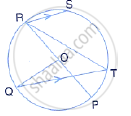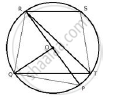Share

The Given Figure Shows a Circle with Centre O Such that Chord Rs is Parallel to Chord Qt, Angle Prt = 20° and Angle Poq = 100°. Calculate: (Iii) Angle Qrs - ICSE Class 10 - Mathematics

ConceptArc and Chord Properties - If Two Arcs Subtend Equal Angles at the Center, They Are Equal, and Its Converse

Question

The given figure shows a circle with centre O such that chord RS is parallel to chord QT, angle PRT = 20° and angle POQ = 100°. Calculate:

(iii) angle QRSSolutionJoin PQ, RQ and ST.

RS ∥ QT
∴ ∠SRT = ∠QTR (alternate angles)
But ∠QTR = 40°
∴ ∠SRT =  40°
Now,
∠QRS =  ∠QRP + ∠PRT + ∠SRT
⇒  ∠QRS = 50° + 20° + 40° = 110°

Is there an error in this question or solution?

APPEARS IN

Solution The Given Figure Shows a Circle with Centre O Such that Chord Rs is Parallel to Chord Qt, Angle Prt = 20° and Angle Poq = 100°. Calculate: (Iii) Angle Qrs Concept: Arc and Chord Properties - If Two Arcs Subtend Equal Angles at the Center, They Are Equal, and Its Converse.
S